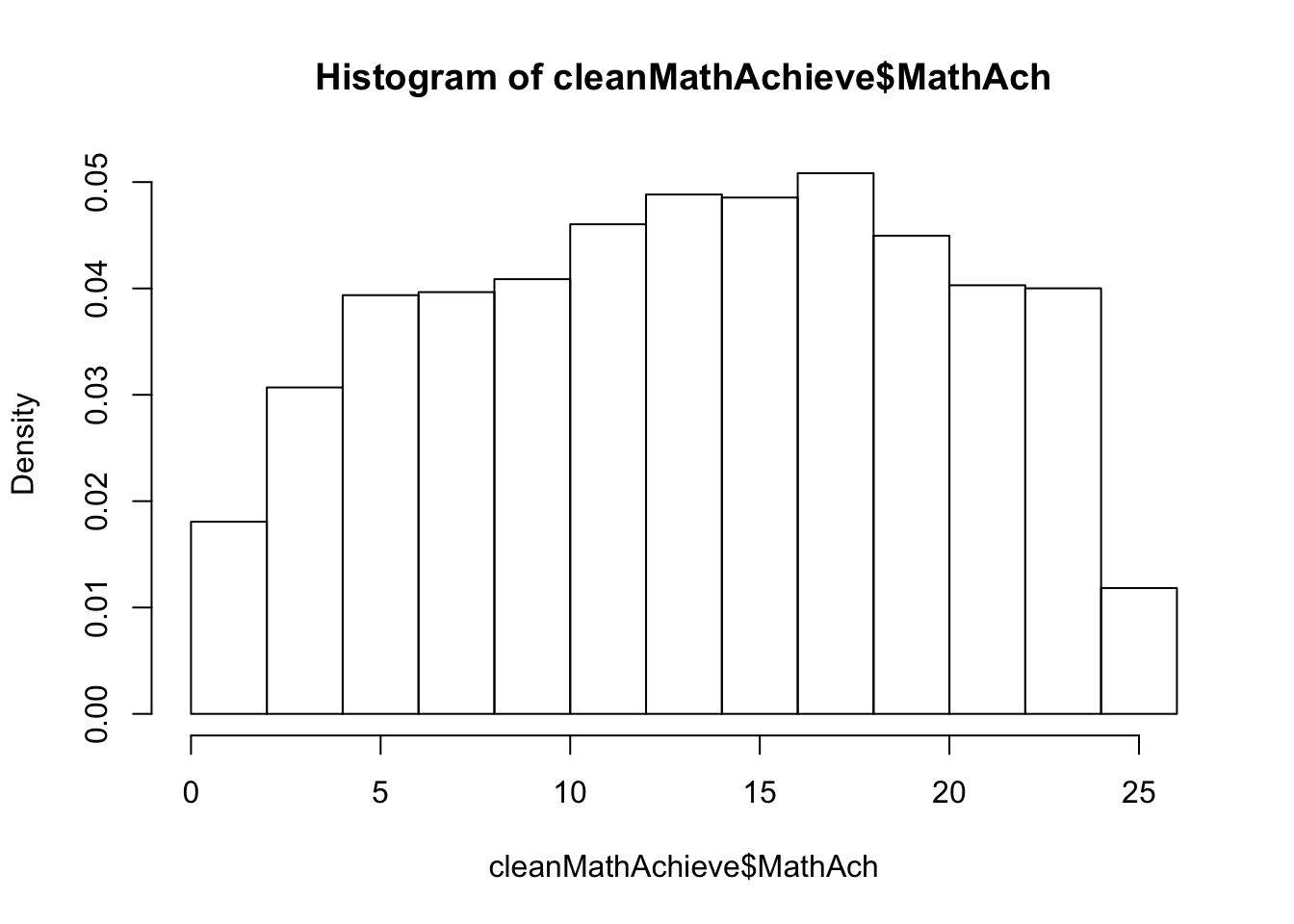``````knitr::opts_chunk\$set(echo = TRUE)
library(MEMSS); library(tidyverse); library(kableExtra); library(INLA); library(Pmisc); library(naniar)
set.seed(12345)
# install.packages("Pmisc", repos="http://R-Forge.R-project.org")

### Introduction

INLA is framework for fast, approximate Bayesian inference. It is especially tailored for hierarchical, latent variable models.

INLA stands for Integrated, Nested, Laplacian Approximation, and it is particularly well suited for models with Gaussian latent variables. If you are interested in fitting generalized hierarchical models with non-normal random effects, as well as other probabilistic models, I encourage you to explore stan.

You can refer to my session slides and its reference for the theoretical and practical background to INLA.

Let’s begin by setting it up in our system (Required: R and RStudio software). INLA is not available in CRAN, so just run the below to download:

``install.packages("INLA", repos=c(getOption("repos"), INLA="https://inla.r-inla-download.org/R/stable"), dep=TRUE)``

As with any other R library, to use it in your work, you have to first call it as

``library(INLA)``

Other useful packages for this workshop, and modern R usage are, `tidyverse`, `kableExtra`, and `ggplot2`. Our dataset can be fetched from the `MEMSS` package and some additional functionalities for reformatting the output of an INLA model can be obtained from `Pmisc`.

### Demo

Let’s jump into a direct application of INLA. We can examine math achievement scores across schools and individuals as a hierarchical regression problem.

Our dataset consists of 7,185 observations depicting the individual math achievement scores (`MathAch`) of children in a metropolitan area, across multiple schools (`School`). In addition, the dataset contains three demographic variables: Binary membership of a minority racial group (`Minority`), binary sex (`Sex`), and numeric socioeconomic status (`SES`).

Research Question: Controlling for sex, socio-economic status, and minority status, what is the role of these fixed effects, as well as that of the school a child attends, with regards to a child’s math achievement scores?

• Bonus: How well can we predict math achievement based on this information?

``````library(MEMSS)
data(MathAchieve)

MathAchieve %>%
kableExtra::kable(digits=3, escape=F, booktab=T,linesep = "") %>%
kable_styling(bootstrap_options = "striped", full_width = F)``````
School Minority Sex SES MathAch MEANSES
1224 No Female -1.528 5.876 -0.428
1224 No Female -0.588 19.708 -0.428
1224 No Male -0.528 20.349 -0.428
1224 No Male -0.668 8.781 -0.428
1224 No Male -0.158 17.898 -0.428
1224 No Male 0.022 4.583 -0.428

To formulate the models and assumptions suitable for this particular dataset, let’s start with some essential EDA (Exploratory Data Analysis).

### EDA

Let’s have a quick look at some summary statistics:

``````MathAchieve %>%
summary() %>%
kableExtra::kable(digits=3, escape=F, booktab=T,linesep = "") %>%
kable_styling(bootstrap_options = "striped", full_width = F)``````
School Minority Sex SES MathAch MEANSES
2305 : 67 No :5211 Female:3795 Min. :-3.758000 Min. :-2.832 Min. :-1.188000
5619 : 66 Yes:1974 Male :3390 1st Qu.:-0.538000 1st Qu.: 7.275 1st Qu.:-0.317000
4292 : 65 NA NA Median : 0.002000 Median :13.131 Median : 0.038000
3610 : 64 NA NA Mean : 0.000143 Mean :12.748 Mean : 0.006138
4042 : 64 NA NA 3rd Qu.: 0.602000 3rd Qu.:18.317 3rd Qu.: 0.333000
8857 : 64 NA NA Max. : 2.692000 Max. :24.993 Max. : 0.831000
(Other):6795 NA NA NA NA NA

Ouch, does it make sense to have negative `MathAch` scores? Negative value on a test score? Let’s clean it up and work with `cleanMathAchieve` going forward.

``````cleanMathAchieve <- subset(MathAchieve, MathAch >= 0)
dim(cleanMathAchieve) # We filtered out 212 records``````
``##  6973    6``

What likelihood should we choose to model our response variable?

``hist(cleanMathAchieve\$MathAch,freq=F)``Let’s look at our features via some pretty dull R base plots:

``````plot(MathAch ~ SES, data=MathAchieve)
plot(MathAch ~ Minority, data=MathAchieve)
plot(MathAch ~ Sex, data=MathAchieve)``````